• +91 9971497814
• info@interviewmaterial.com

# Chapter 6- बीजीय व्यंजक Ex-6-e Interview Questions Answers

### Related Subjects

Question 1 : निम्नांकित के मान ज्ञात कीजिए, यदि x = 7, y = 3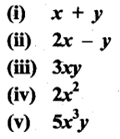Answer 1 :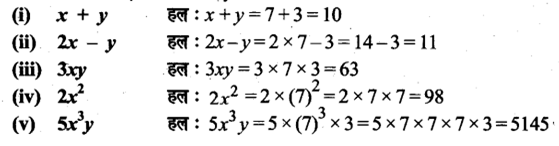Question 2 : सही विकल्प छाँटिए (अँटकर)-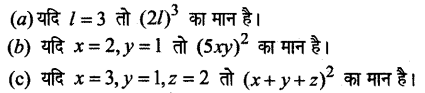Answer 2 :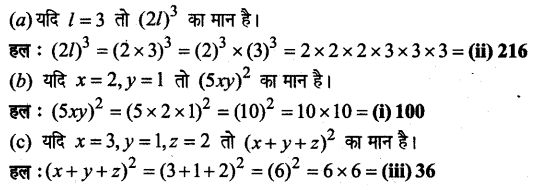Question 3 : यदि x = 4 तथा y = 3 तो पार्श्व चित्र में आयत की भुजाएँ ज्ञात कीजिए।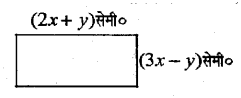आयत की लं० = (2x + y) = (2 x 4 + 3) = 8 + 3 = 11 सेमी०
आयते की चौ० = (3x – y) = (3 x 4 – 3) = 12 – 3 = 9 सेमी०

Question 4 : यदि a = 4 तो पाश्र्व चित्र में त्रिभुज की भुजाएँ ज्ञात कीजिए।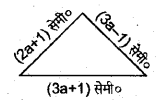त्रिभुज की भुजाएँ = (2a + 1) सेमी०, (3a – 1) सेमी० तथा (3a + 1) सेमी०
= (2 x 4 + 1) सेमी०, (3 x 4 – 1) सेमी० तथा (3 x 4 + 1) सेमी०
= (8 + 1) सेमी०, (12 – 1) सेमी० तथा (12 + 1) सेमी०
= 9 सेमी०, 11 सेमी० तथा 13 सेमी०

Question 5 : यदि y = -1 तो बीजीय व्यंजक 2y2 + 3y2 + y – 3 का मान ज्ञात कीजिए।

2y2 + 3y2 +y – 3
= 2 x (-1)2 + 3(-1)2 + (-1) – 3
= 2 x 1 + 3 x 1 – 1 – 3
= 2 + 3 – 4
= 5 – 4
= 1

Question 6 : यदि a= -2, b = 2 तथा c = 1तो बीजीय व्यंजक 4a3 – 2abc+ 3bc + b3 का मान ज्ञात कीजिए।

4(-2)3 –2(-2) x 2 x 1 + 3 x 2 x 1 + (2)3
= 4(-8) + 8 + 6 + 4
= -32 + 8 + 6 + 4
= -32 + 18
= -14

Question 7 : यदि a= 3 तथा b = 2 तो निम्नांकित को सत्यापित कीजिए।
(i) (a + b)2 = a2 + 2ab + b2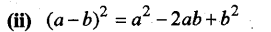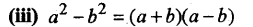Answer 7 : (i) (a + b)2 = a2 + 2ab + b2
(a + b)2 = a2 + 2ab + b2
में a और b का मान रखने पर
(3 + 2)2 = (3)2 + 2 x 3 x 2 + (2)2
(5)2 = 9 + 12 + 4
25 = 25
L.H.S. = R.H.S.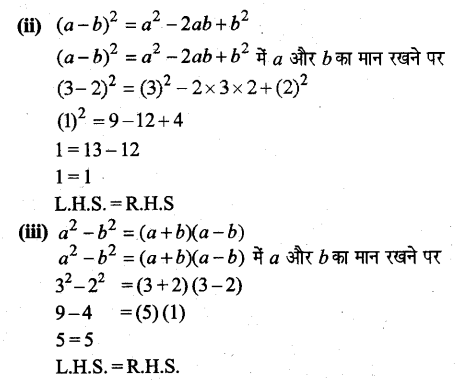Todays Deals### Chapter 6- बीजीय व्यंजक Ex-6-e Contributorskrishan

Name:
Email:

# Latest News# 9000 interview questions in different categories